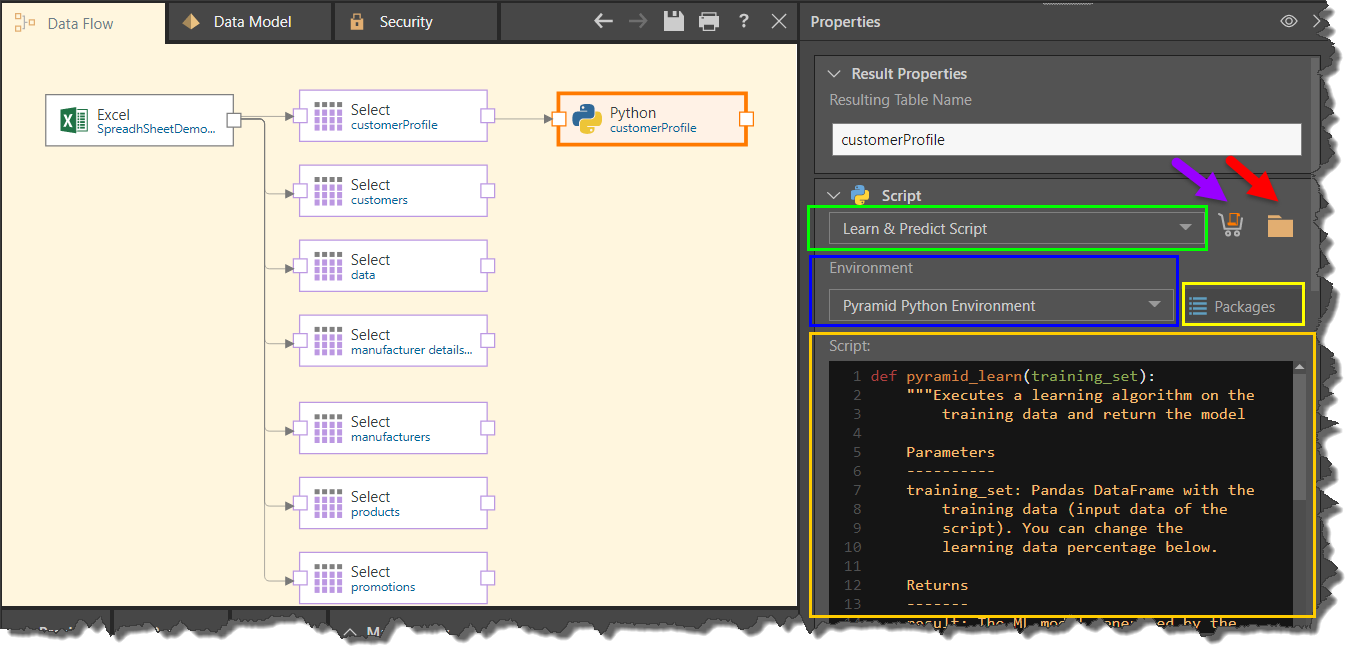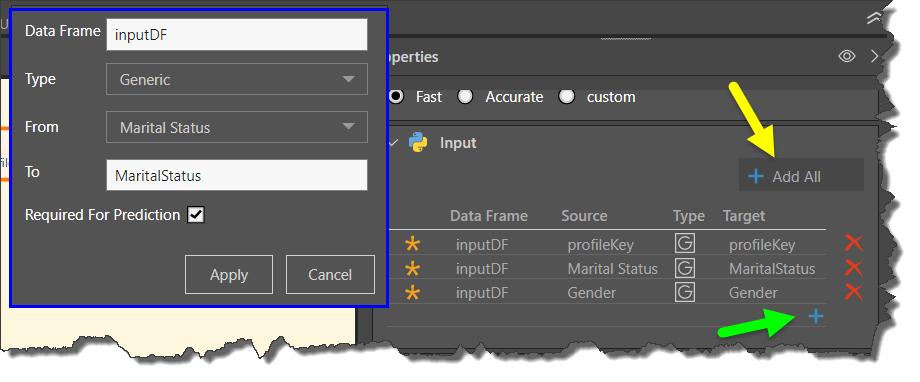# Learn and Predict Scripts

Learn and predict algorithms can be saved to produce machine learning (ML) models. The ML model can then be saved, set as a target, and downloaded. This powerful tool is then used to make predictions based on your data set. For instance, you might want to know the likelihood of a purchase based on a set of criteria about a customer - say, age, address, and income.

The learn and predict algorithm can be added to a Python or R scripting node in a few ways: it can be written or pasted into the script window; you can download an algorithm from the Pyramid Marketplace; or you can select a learn and predict script that has been saved to Pyramid's content management system.

## Configure the Scripting Node

Be sure to select Learn & Predict Script as the script type (green highlight below):### Running Process Type

Scroll down below the script window to continue configuring the Python node. This selection determines the amount of data that is used to train the algorithm (green highlight in the image above).

Fast: uses 20% of the data.

Accurate: uses 90% of the data.

Custom: enter a custom amount.### Input

When configuring the input columns, determine whether or not each column is required for the prediction.### Model Score

The model score is evaluated after the algorithm has been run. The score that the algorithm assigns to the ML model, which indicates how reliable the model is (green highlight in the image above). To produce this score, the algorithm compares its predictions based on the training data with the actual data.

### Save ML Model

Here you have the option to name and save the model, and to set it as a target in the ETL (yellow highlight above).

Save Model: save the algorithm output as a machine learning model (see below to learn more).

Set as Target: set the Python node as the target in the data flow (see below to learn more).

## Learn and Predict Algorithm

The learn and predict algorithm must contain the following three parameters:

### Learn Function

def pyramid_learn(training_set):

Write a learn function, which will take the training data (input) and return the Machine Learning model (output).

To determine the size of the training data, make a selection from the Running Process Type below the Script window.

### Eval Function

def pyramid_eval(model, testing_set):

Write a Pyramid eval function. The eval function evaluates the ML model produced by the learn function against a testing set (this is not the same testing set that was used by the learn function). It returns a model score indicating the reliability of the predictions, which is displayed in the 'Model Score' panel.

The eval function may or may not contain a predict function. It is generally used for prediction and computation.

### Predict Function

def pyramid_predict(model, df):

Write a predict function which will apply the ML model to the entire data set. The output of the predict function is a Pandas DataFrame with prediction results. The output may be added as columns to an existing table, or used to create a new table.

### PMML Function

def pyramid_toPmml(model):

Optionally, write an export function which serializes the model to PMML format in order to use the Pyramid-trained models in other platforms. The exported PMML is available for download through the Admin console’s Data Sources tab.

## Save ML Model

Select this option to save the algorithm's output as a machine learning model. This stores the existing results and allows you to add the ML model to another data flow later on; this is useful if you want to apply the ML model to new data in the data flow. In this scenario, the algorithm will run faster because the previous results are stored. As the learn function was already run on the algorithm, only the predict function will run.